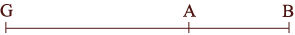SEARCH HOMEMath Central Quandaries & QueriesQuestion from Matthew, a parent: "G", "A" and "B" are collinear. "A" is between "G" and "B". "GA" Is 14 less than 3 times "AB". "GB"=46 Find "GA" How would you solve this?Hi Matthew,

The fact that G, A and B are collinear means that they lie on a line. I drew the segment of the line between G and B.GA is the distance from G to A, AB is the distance from A to B and GB is the distance from G to B.

"GA Is 14 less than 3 times AB"

Write "3 times AB" as a mathematical expression. What is 14 less than this quantity? This is GA so you have an equation relating GA and AB.

Look at the diagram. Write a mathematical expression that relates GA, AB and GB. But GB = 46 so this gives you a second equation relating GA and AB.

Solve these two equations for GA.

Write back if you need more assistance.

PennyMath Central is supported by the University of Regina and The Pacific Institute for the Mathematical Sciences.